# The Boltzmann Equation, Two-Term Approximation Interface

April 8, 2015

In a previous blog post, we introduced readers to different kinds of electron energy distribution functions (EEDFs) and their importance in plasma modeling. Today, we focus our attention on the Boltzmann Equation, Two-Term Approximation interface, demonstrating its use with an example from our Model Library.

### An Introduction to the Boltzmann Equation, Two-Term Approximation Interface

In a plasma model, the electron energy distribution function as well as the transport properties of the electrons (e.g., electron mobility) are needed. For the simplest cases, a Maxwellian EEDF and a constant value for the electron mobility can be used. The other transport properties are then computed in COMSOL Multiphysics using Einstein’s relation. However, in some cases, it might be advantageous to use a more elaborate form like a Boltzmann EEDF and define the electron transport properties as a function of the electron energy. But how do we obtain this data?

The answer: The Boltzmann Equation, Two-Term Approximation interface in COMSOL Multiphysics. Examples illustrating the use of this interface are available in the Model Library, one of which is the Argon Boltzmann Analysis model. To compute the Boltzmann EEDF, parameters such as the ionization degrees of the plasma are required. These parameters are not known a priori. Thus, the procedure is an iterative process.

The process begins with making an initial guess for the parameters and solving the Boltzmann equation. Then, the EEDF and transport properties are imported, if needed, to your plasma model. Finally, the plasma model is computed and the Boltzmann equation is resolved with the new volume-averaged parameters from the plasma model. You can continue to repeat these steps until convergence is reached.

Let’s walk through the steps of importing the data into the plasma model.

### Electron Energy Distribution Function and Electron Transport Properties

The figure below shows a Boltzmann EEDF in an argon plasma. The plasma features a gas temperature of 400 K, an electron density of 1016 1/m³, an ionization degree of 10-10, and a mole fraction of excited argon of 0.01. It can be observed that the EEDF is a function of the electron energy. Normally, an array of curves for the mean electron energy as a parameter is required.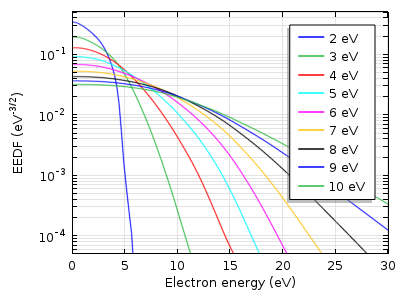Boltzmann EEDF in an argon plasma.

The next figure depicts the corresponding reduced electron transport properties computed with the Boltzmann Equation, Two-Term Approximation interface. The data is a function of the mean electron energy.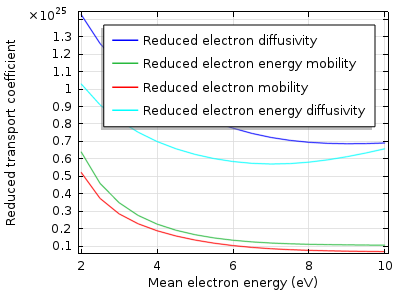Reduced electron transport properties in an argon plasma.

#### Exporting the Data from the Boltzmann Equation, Two-Term Approximation Interface

The EEDF has to be imported to the plasma model as a spreadsheet consisting of three rows. The first row (x-data) has to be the electron energy (eV), while the second row (y-data) has to be the mean electron energy (eV). Meanwhile, the third row must contain the value for the distribution function (eV^(-3/2)). Finally, you need to export a 2D plot that looks like the following image.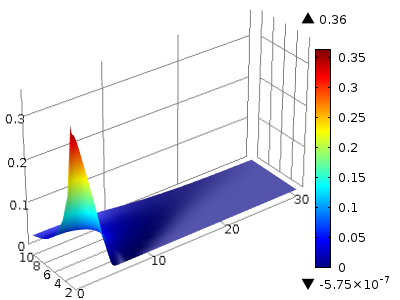A 2D plot of the Boltzmann EEDF. Here, the x-axis indicates the electron energy and the y-axis represents the mean electron energy. The color illustrates the value of the distribution function.

To export the EEDF in the desired format, use the Parametric Extrusion data set. Right-click “Data Sets” and choose Parametric Extrusion 1D. Set the level scale factor to 1. Then, right-click the Parametric Extrusion 1D data and choose Add Data to Export. Select a file name and click “Export”.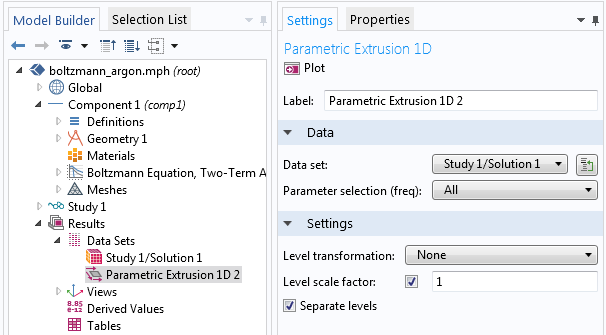The transport properties can be exported in a straightforward manner from the respective 1D plot. Right-click the Global data and choose Add Plot Data to Export. Select a file name and click “Export”.

#### Importing the Data to the Plasma Model

To import the EEDF to the plasma model, create an Interpolation function. Select File as the Data source and enter “2” in the Number of arguments field. Click “Browse” and import the file.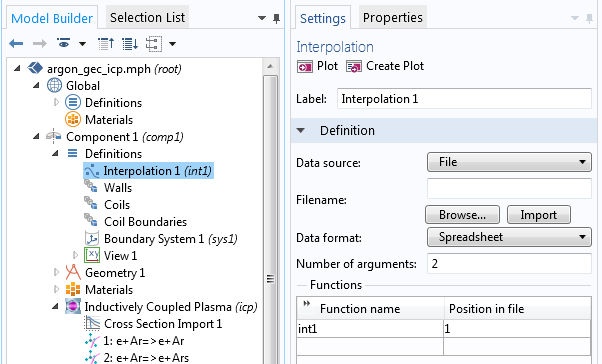Once you have done this, you can choose the interpolation function as an EEDF in the Electron Energy Distribution Function Settings in the main node of the plasma model. This is illustrated in the screenshot below.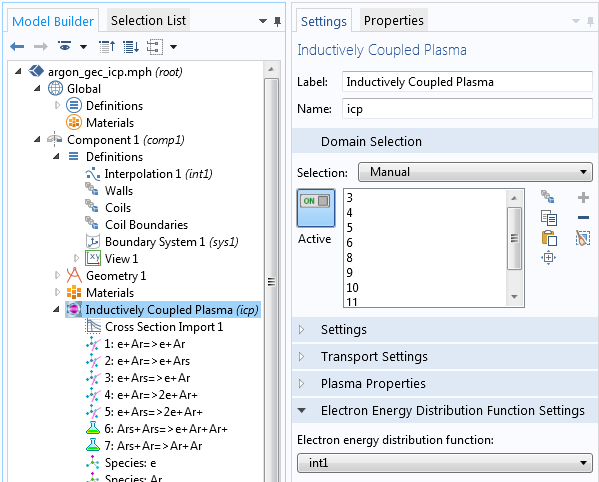The functions for the transport properties, such as electron mobility and diffusivity, can also be imported as an interpolation function. Here, however, the number of arguments is 1. In the plasma model node, you can use this interpolation function by entering “int2(icp.ebar)”. In this case, int2 is the name of the function, icp is the tag of the interface, and ebar is the mean electron energy.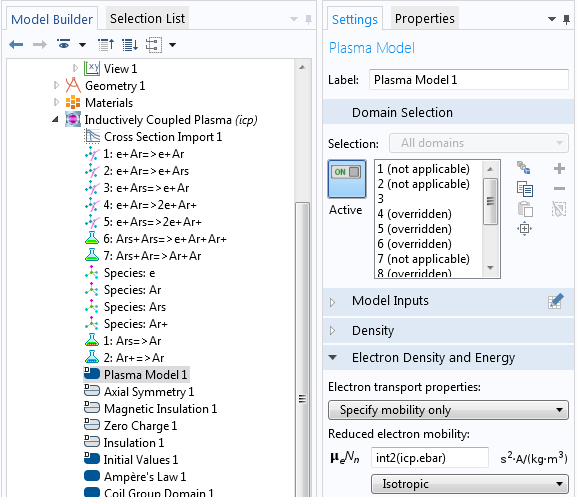#### Comentários (4)

##### Faça um Comentário
Carregando...##### CHANYEOP PARK
January 14, 2016

Can anyone tell me what 3 0(in the 3rd line) and 1. 1.(in the 4th line) means in the ‘collision cross section’ file for the plasma module?

EXCITATION
e+O2=>e+O2
0.1900 3 0
1. 1.
—————————
4.000000e+000 2.220446e-036

4.500000e+001 2.220446e-036
—————————##### Pouyanesh Co.
October 14, 2020

3 is the statistical weight ratio and 0 means the unbalance relation between reaction and the reversed reaction.##### weifeng deng
December 7, 2019##### weifeng deng
December 7, 2019

Can I share the file of interpolation function?

EXPLORE O BLOG COMSOL